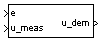# Self-Conditioned [A,B,C,D]

Implement state-space controller in self-conditioned form

• Library:
• Aerospace Blockset / GNC / Control

•## Description

The Self-Conditioned [A,B,C,D] block can be used to implement the state-space controller defined by

`$\left[\begin{array}{l}\stackrel{˙}{x}=Ax+Be\\ u=Cx+De\end{array}\right]$`

in the self-conditioned form

`$\begin{array}{l}\stackrel{˙}{z}=\left(A-HC\right)z+\left(B-HD\right)e+H{u}_{meas}\\ {u}_{dem}=Cz+De\end{array}$`

The input ${u}_{meas}$ is a vector of the achieved actuator positions, and the output ${u}_{dem}$ is the vector of controller actuator demands. In the case that the actuators are not limited, then ${u}_{meas}={u}_{dem}$ and substituting the output equation into the state equation returns the nominal controller. In the case that they are not equal, the dynamics of the controller are set by the poles of A-HC.

Hence H must be chosen to make the poles sufficiently fast to track ${u}_{meas}$ but at the same time not so fast that noise on e is propagated to ${u}_{dem}$. The matrix H is designed by a callback to the Control System Toolbox™ command `place` to place the poles at defined locations.

## Limitations

This block requires the Control System Toolbox license.

## Ports

### Input

expand all

Control error, specified as a vector.

Data Types: `double`

Achieved actuator positions, specified as a vector.

Data Types: `double`

### Output

expand all

Actuator demands, specified as a vector.

Data Types: `double`

## Parameters

expand all

A-matrix of the state-space implementation. The A-matrix should have three dimensions, the last one corresponding to the scheduling variable v. For example, if the A-matrix corresponding to the first entry of v is the identity matrix, then ```A(:,:,1) = [1 0;0 1];```.

#### Programmatic Use

 Block Parameter: `Ak` Type: character vector Values: vector Default: ```'[-1 -0.2;0 -3]'```

B-matrix of the state-space implementation, specified as a array. The B-matrix should have three dimensions, the last one corresponding to the scheduling variable v. For example, if the B-matrix corresponding to the first entry of v is the identity matrix, then ```B(:,:,1) = [1 0;0 1];```.

#### Programmatic Use

 Block Parameter: `Bk` Type: character vector Values: vector Default: `'[1;1]'`

C-matrix of the state-space implementation, specified as a array. The C-matrix should have three dimensions, the last one corresponding to the scheduling variable v. For example, if the C-matrix corresponding to the first entry of v is the identity matrix, then ```C(:,:,1) = [1 0;0 1];```.

#### Programmatic Use

 Block Parameter: `Ck` Type: character vector Values: vector Default: ```'[1 0]'```

D-matrix of the state-space implementation. The D-matrix should have three dimensions, the last one corresponding to the scheduling variable v. For example, if the D-matrix corresponding to the first entry of v is the identity matrix, then `D(:,:,1) = [1 0;0 1];`.

#### Programmatic Use

 Block Parameter: `Dk` Type: character vector Values: vector Default: `'0.02'`

Initial states for the controller, that is, initial values for the state vector, z. It should have length equal to the size of the first dimension of A.

#### Programmatic Use

 Block Parameter: `x_initial` Type: character vector Values: vector Default: `'0'`

Desired poles of A-H*C, specified as a vector. Hence the number of pole locations defined should be equal to the dimension of the A-matrix.

#### Programmatic Use

 Block Parameter: `vec_w` Type: character vector Values: vector Default: ```'[-5 -2]'```

expand all

 Kautsky, Nichols, and Van Dooren, "Robust Pole Assignment in Linear State Feedback," International Journal of Control, Vol. 41, Number 5, 1985, pp. 1129-1155.

## SupportGet trial now